### Balance Equations: Mass Balance

Let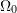and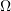 represent the reference and the deformed configurations, respectively, of a body embedded in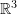. Let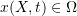represent the image of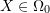at a certain point in time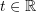under the bijective smooth mapping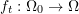. Notice that the setwhich is the range of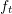changes as time changes. The velocity vector is described by the vector valued function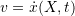.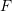and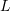are the deformation and the velocity gradients, respectively, while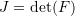. The density distribution of the continuum body in the reference configuration is given by the function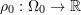. As the body deforms, the density at each material point changes with time and thus can be described by a function of both time and material points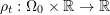. The spatial density distribution of the continuum body in the deformed configuration is a function of the positionand of timeand is described by the function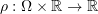. The difference between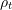and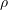is merely a change of coordinates from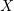to: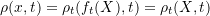In this section, we will use the physical law of mass balance to establish the relationship between the mass densities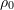,and. If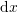and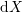represent an infinitesimal volume in the deformed and reference configurations, respectively, then, the relationship between the volume in the deformed versus the reference configurations is given by: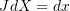Ifis the actual mass of both infinitesimal volumes and assuming that mass is preserved then the relationship between the densities is given by: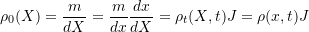This relationship can also be obtained using integrals over the whole continuum body as follows. The total mass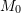of the reference configuration can be calculated by the integral: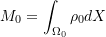The total mass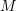in the deformed configuration can be calculated by the integral: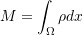The volume integral overcan be replaced by the volume integral overby using the relationship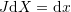: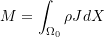Assuming that the total mass is conserved, i.e.,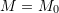, and sinceis arbitrary, the relationship between the densities is retrieved: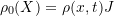### The Continuity Equation

The continuity equation describes the rates of change of the density functions. We first recall the relationship between the velocity gradientand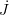obtained in the velocity gradient section: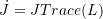Assuming preservation of mass, i.e., the rate of change of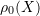is equal to zero leads to the following equivalent forms of the continuity equation:

#### Lagrangian Formulation

The continuity equation as a function ofis given by: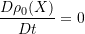Alternatively, the continuity equation as a function of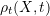is given by: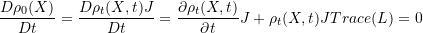Since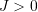we reach the following form of the continuity equation: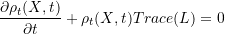#### Eulerian Formulation

The continuity equation as a function of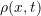is given by: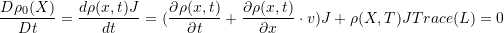Sincewe reach the following form of the continuity equation: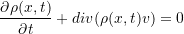where, according to the results in the vector calculus section: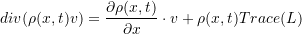#### Continuity Equation of Fluids

In fluids, the Eulerian formulation is adopted along with the approximation that the fluid is incompressible, i.e.,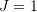and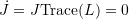. Therefore, the continuity equation reduces to: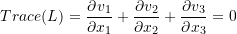#### Continuity Equation for Growth or Mass Transfer

In problems where there is growth or mass transfer, the density in the reference configuration is assumed to vary in time and its rate of change is equal to the source or the sink of the local mass change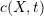and the continuity equation is then derived from the equation: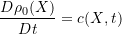#### Example

The position function of a 2units by 2units plate has the form: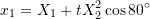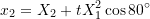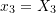where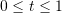units. The density of the material in the reference configuration at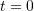is given by the function: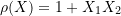The following tool draws the contour plots ofand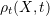in the reference configuration and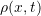in the deformed configuration as a function of time. Vary the time to see the effect on the deformation and the effect on the density plots. Note thatdoes not change when time evolves. The computations are based on first calculating the deformation gradient as follows: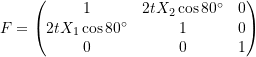thus, the determinant ofis given by: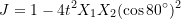The densityas time evolves is equal to: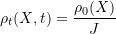Findingrequires solving a nonlinear set of equations to expressin terms of.

#### Problem

The two dimensional displacement field of a 2 units by 3 units plate is given by: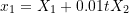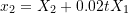If the density in the reference configuration is given by the function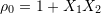units of density, find the expressions forand. For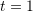, draw the contour plots of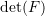,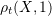, and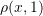.

–>